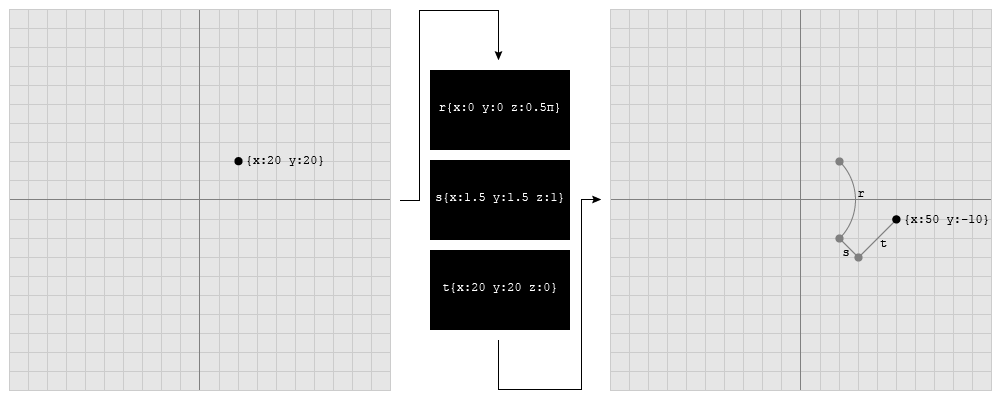# Into Vertex Shaders Addendum 1: Matrix Math and You

## Representation in Code

`transformedVector = vector * transformationMatrix;`
`var vector = new THREE.Vector3(20, 20, 0);var matrix = new THREE.Matrix4();matrix.makeTranslation(10, 40, 0);vector.applyMatrix4(matrix);`
`matrix.makeRotationX(angle);matrix.makeRotationY(angle);matrix.makeRotationZ(angle);matrix.makeRotationAxis(axis, angle);matrix.makeRotationFromEuler(euler);matrix.makeRotationFromQuaternion(quaternion);`
`matrix.makeRotationX(Math.PI);matrix.makeRotationAxis(new THREE.Vector3(1, 0, 0), Math.PI);`
`var translation = new THREE.Vector3();var rotation = new THREE.Quaternion();var scale = new THREE.Vector3();var matrix = new THREE.Matrix4();matrix.compose(translation, rotation, scale);`

## Stacking boxesRotation (r), Scale (s) and Translation (t).
`combinedMatrix = rotationMatrix * scaleMatrix * translationMatrix;`
`var rotationMatrix = new THREE.Matrix4().makeRotation(...);var scaleMatrix = new THREE.Matrix4().makeScale(...);var translationMatrix = new THREE.Matrix4().makeTranslation(...);var combinedMatrix = new THREE.Matrix4();combinedMatrix.multiply(rotationMatrix);combinedMatrix.multiply(scaleMatrix);combinedMatrix.multiply(translationMatrix);`

## Pre-multiplication and Post-multiplication

`1 * 2 * 3 * 4 = 244 * 3 * 2 * 1 = 24`
`// start with the transformation for this nodevar matrixWorld = new THREE.Matrix4().copy(node.matrix);// get the first parentvar parent = node.parent;do {  // pre-multiply  matrixWorld.multiplyMatrices(parent.matrix, matrixWorld);  // get next parent  parent = parent.parent;}while (parent);`
`4 * 5 = 20;20 / 5 = 4;`
`transformedVector = originalVector * matrix;inverseMatrix = matrix.inverse();untransformedVector = transformedVector * inverseMatrix;// untransformedVector == originalVector`
`var matrix = new THREE.Matrix4();// apply transformation ...var inverseMatrix = new THREE.Matrix4();matrix.getInverse(inverseMatrix);`